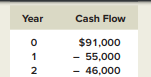### Create an Account

Home / Questions / Calculating IRR A project has the following cash flows What is the IRR for this project...

# Calculating IRR A project has the following cash flows What is the IRR for this project If the required return is 10 percent should the firm accept the project What is the NPV of this project

Calculating IRR. A project has the following cash flows:What is the IRR for this project? If the required return is 10 percent, should the firm accept the project? What is the NPV of this project? What is the NPV of the project if the required return is 0 percent? 24 percent? What is going on here? Sketch the NPV profile to help you with your answer

May 25 2020 View more View LessSubscribe To Get Solution